Home
Hostname: page-component-684899dbb8-489z4 Total loading time: 0.403 Render date: 2022-05-29T08:06:55.170Z Has data issue: true Feature Flags: { "shouldUseShareProductTool": true, "shouldUseHypothesis": true, "isUnsiloEnabled": true, "useRatesEcommerce": false, "useNewApi": true }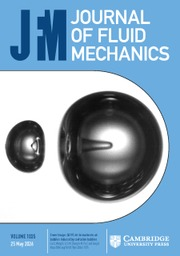Journal of Fluid Mechanics

# Two-dimensional magnetohydrodynamic turbulence in the limits of infinite and vanishing magnetic Prandtl number

Published online by Cambridge University Press:  14 May 2013

*

## Abstract

HTML view is not available for this content. However, as you have access to this content, a full PDF is available via the ‘Save PDF’ action button.

We study both theoretically and numerically two-dimensional magnetohydrodynamic turbulence at infinite and zero magnetic Prandtl number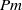$\mathit{Pm}$ (and the limits thereof), with an emphasis on solution regularity. For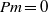$\mathit{Pm}= 0$, both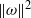$\Vert \omega \Vert ^{2}$ and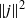$\Vert j\Vert ^{2}$, where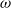$\omega$ and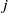$j$ are, respectively, the vorticity and current, are uniformly bounded. Furthermore,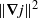$\Vert \boldsymbol{\nabla} j\Vert ^{2}$ is integrable over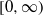$[0, \infty )$. The uniform boundedness of$\Vert \omega \Vert ^{2}$ implies that in the presence of vanishingly small viscosity$\nu$ (i.e. in the limit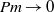$\mathit{Pm}\rightarrow 0$), the kinetic energy dissipation rate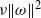$\nu \Vert \omega \Vert ^{2}$ vanishes for all times$t$, including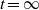$t= \infty$. Furthermore, for sufficiently small$\mathit{Pm}$, this rate decreases linearly with$\mathit{Pm}$. This linear behaviour of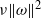$\nu \Vert \omega \Vert ^{2}$ is investigated and confirmed by high-resolution simulations with$\mathit{Pm}$ in the range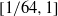$[1/ 64, 1]$. Several criteria for solution regularity are established and numerically tested. As$\mathit{Pm}$ is decreased from unity, the ratio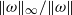$\Vert \omega \Vert _{\infty } / \Vert \omega \Vert$ is observed to increase relatively slowly. This, together with the integrability of$\Vert \boldsymbol{\nabla} j\Vert ^{2}$, suggests global regularity for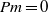$\mathit{Pm}= 0$. When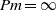$\mathit{Pm}= \infty$, global regularity is secured when either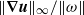$\Vert \boldsymbol{\nabla} \boldsymbol{u}\Vert _{\infty } / \Vert \omega \Vert$, where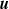$\boldsymbol{u}$ is the fluid velocity, or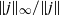$\Vert j\Vert _{\infty } / \Vert j\Vert$ is bounded. The former is plausible given the presence of viscous effects for this case. Numerical results over the range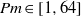$\mathit{Pm}\in [1, 64]$ show that$\Vert \boldsymbol{\nabla} \boldsymbol{u}\Vert _{\infty } / \Vert \omega \Vert$ varies slightly (with similar behaviour for$\Vert j\Vert _{\infty } / \Vert j\Vert$), thereby lending strong support for the possibility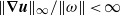$\Vert \boldsymbol{\nabla} \boldsymbol{u}\Vert _{\infty } / \Vert \omega \Vert \lt \infty$ in the limit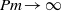$\mathit{Pm}\rightarrow \infty$. The peak of the magnetic energy dissipation rate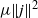$\mu \Vert j\Vert ^{2}$ is observed to decrease rapidly as$\mathit{Pm}$ is increased. This result suggests the possibility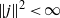$\Vert j\Vert ^{2} \lt \infty$ in the limit$\mathit{Pm}\rightarrow \infty$. We discuss further evidence for the boundedness of the ratios$\Vert \omega \Vert _{\infty } / \Vert \omega \Vert$,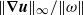$\Vert \boldsymbol{\nabla} \boldsymbol{u}\Vert _{\infty } / \Vert \omega \Vert$ and$\Vert j\Vert _{\infty } / \Vert j\Vert$ in conjunction with observation on the density of filamentary structures in the vorticity, velocity gradient and current fields.

## JFM classification

Type
Papers
Information
Journal of Fluid Mechanics , 25 June 2013 , pp. 195 - 215
Creative Commons## References

Beale, J. T., Kato, T. & Majda, A. 1984 Remarks on the breakdown of smooth solutions for the 3-D Euler equations. Commun. Math. Phys. 94, 6164.CrossRefGoogle Scholar
Beresnyak, A. 2011 Spectral slope and Kolmogorov constant of MHD turbulence. Phys. Rev. Lett. 106, 075001.CrossRefGoogle ScholarPubMed
Blackbourn, L. A. K. & Tran, C. V. 2012 On energetics and inertial range scaling laws of two-dimensional magnetohydrodynamic turbulence. J. Fluid Mech. 703, 238254.CrossRefGoogle Scholar
Brandenburg, A. 2011a Nonlinear small-scale dynamos at low magnetic Prandtl numbers. Astrophys. J. 741, 92.CrossRefGoogle Scholar
Brandenburg, A. 2011b Dissipation in dynamos at low and high magnetic Prandtl numbers. Astron. Nachr. 332, 5156.CrossRefGoogle Scholar
Caflisch, R. E., Klapper, I. & Steel, G. 1997 Remarks on singularities, dimension, and energ dissipation for ideal hydrodynamics MHD. Commun. Math. Phys. 184, 443455.CrossRefGoogle Scholar
Cao, C. & Wu, J. 2011 Global regularity for the 2D MHD equations with mixed partial dissipation and magnetic diffusion. Adv. Math. 226, 18031822.CrossRefGoogle Scholar
Chambers, K. & Forbes, L. K. 2011 The magnetic Rayleigh–Taylor instability for inviscid and viscous fluids. Phys. Plasmas 18, 052101.CrossRefGoogle Scholar
Dritschel, D. G. & Tobias, S. M. 2012 Two-dimensional magnetohydrodynamic turbulence in the small magnetic Prandtl limit. J. Fluid Mech. 703, 8598.CrossRefGoogle Scholar
Dritschel, D. G., Tran, C. V. & Scott, R. K. 2007 Revisiting Batchelor’s theory of two-dimensional turbulence. J. Fluid Mech. 591, 379391.CrossRefGoogle Scholar
Galtier, S., Pouquet, A. & Mangeney, A. 2005 Spectral scaling laws for incompressible anisotropic magnetohydrodynamic turbulence. Phys. Plasmas 12, 092310.CrossRefGoogle Scholar
Goldreich, P. & Sridhar, S. 1995 Toward a theory of interstellar turbulence. II. Strong Alfvénic turbulence. Astrophys. J. 438, 763775.CrossRefGoogle Scholar
Iroshnikov, P. S. 1964 Turbulence of a conducting fluid in a strong magnetic field. Sov. Astron. 7, 566571.Google Scholar
Iskakov, A. B., Schekochihin, A. A., Cowley, S. C., McWilliam, J. C. & Proctor, M. R. E. 2007 Numerical demonstration of fluctuation dynamo at low magnetic Prandtl numbers. Phys. Rev. Lett. 98, 208501.CrossRefGoogle ScholarPubMed
John, F. & Nirenberg, L. 1961 On functions of bounded mean oscillation. Commun. Pure Appl. Maths 14, 415426.CrossRefGoogle Scholar
Kiselev, A., Nazarov, F. & Volberg, A. 2007 Global well-posedness for the critical 2D dissipative quasi-geostrophic equation. Invent. Math. 167, 445453.CrossRefGoogle Scholar
Kozono, H. & Tanuichi, Y. 2000 Limiting case of the Sobolev inequality in BMO, with application to the Euler equations. Commun. Math. Phys. 214, 191200.CrossRefGoogle Scholar
Kraichnan, R. H. 1965 Inertial-range spectrum of hydromagnetic turbulence. Phys. Fluids 8, 13851387.CrossRefGoogle Scholar
Lei, Z. & Zhou, Y. 2009 BKM’s criterion and global weak solutions for magnetohydrodynamics with zero viscosity. Discrete Contin. Dyn. Syst. 25, 575583.Google Scholar
Moffatt, K. H. 1967 On the suppression of turbulence by a uniform magnetic field. J. Fluid Mech. 28, 571592.CrossRefGoogle Scholar
Ng, C. S., Bhattacharjee, A., Munsi, D., Isenberg, P. A. & Smith, C. W. 2010 Kolmogorov versus Irosnikov–Kraichnan spectra: consequence for ion heating in the Solar wind. J. Geophys. Res. 115, A02101.CrossRefGoogle Scholar
Nirenberg, L. 1959 On elliptic partial differential equations. Ann. Scuola Norm. Sup. Pisa 13, 115162.Google Scholar
Orszag, S. A. & Tang, C.-M. 1979 Small-scale structure of two-dimensional magnetohydrodynamic turbulence. J. Fluid Mech. 90, 129143.CrossRefGoogle Scholar
Pouquet, A. 1978 On two-dimensional magnetohydrodynamic turbulence. J. Fluid Mech. 88, 116.CrossRefGoogle Scholar
Sridhar, S. & Goldreich, P. 1994 Toward a theory of interstellar turbulence. I. Weak Alfvénic turbulence. Astrophys. J. 432, 612621.CrossRefGoogle Scholar
Stein, E. M. 1970 Singular Integrals and Differentiability Properties of Functions. Princeton University Press.Google Scholar
Stein, E. M. 1993 Harmonic Analysis: Real-variable Methods, Orthogonality, and Oscillatory Integrals. Princeton University Press.Google Scholar
Tobias, S. M. & Cattaneo, F. 2008 Dynamo action in complex flows: the quick and the fast. J. Fluid Mech. 601, 101122.CrossRefGoogle Scholar
Tran, C. V. & Blackbourn, L. A. K. 2012 A dynamical systems approach to fluid turbulence. Fluid Dyn. Res. 44, 031417.CrossRefGoogle Scholar
Tran, C. V., Blackbourn, L. A. K. & Scott, R. K. 2011 Number of degrees of freedom and energy spectrum of surface quasi-geostrophic turbulence. J. Fluid Mech. 684, 427440.CrossRefGoogle Scholar
Tran, C. V. & Dritschel, D. G. 2006 Vanishing enstrophy dissipation in two-dimensional Navier–Stokes turbulence in the inviscid limit. J. Fluid Mech. 559, 107116.CrossRefGoogle Scholar
Tran, C. V. & Yu, X. 2012 Bounds for the number of degrees of freedom of magnetohydrodynamics turbulence in two and three dimensions. Phys. Rev. E 85, 066323.CrossRefGoogle Scholar
Tran, C. V., Yu, X. & Zhai, Z. 2013a On global regularity of 2D generalized magnetohydrodynamic equations. J. Differential Equations 254, 41944216.CrossRefGoogle Scholar
Tran, C. V., Yu, X. & Zhai, Z. 2013b Note on solution regularity of the generalized magnetohydrodynamic equations with partial dissipation. Nonlinear Anal. 85, 4351.CrossRefGoogle Scholar
Verma, M. K., Roberts, D. A., Goldstein, M. L., Gosh, S. & Stribling, W. T. 1996 A numerical study of the nonlinear cascade of energy in magnetohydrodynamic turbulence. J. Geophys. Res. 101, 2161921625.CrossRefGoogle Scholar
Wu, J. 2011 Global regularity for a class of generalized magnetohydrodynamic equations. J. Math. Fluid Mech. 13, 295305.CrossRefGoogle ScholarYou have AccessOpen access
5
Cited by

# Save article to Kindle

Note you can select to save to either the @free.kindle.com or @kindle.com variations. ‘@free.kindle.com’ emails are free but can only be saved to your device when it is connected to wi-fi. ‘@kindle.com’ emails can be delivered even when you are not connected to wi-fi, but note that service fees apply.

Find out more about the Kindle Personal Document Service.

Two-dimensional magnetohydrodynamic turbulence in the limits of infinite and vanishing magnetic Prandtl number
Available formats
×

# Save article to Dropbox

To save this article to your Dropbox account, please select one or more formats and confirm that you agree to abide by our usage policies. If this is the first time you used this feature, you will be asked to authorise Cambridge Core to connect with your Dropbox account. Find out more about saving content to Dropbox.

Two-dimensional magnetohydrodynamic turbulence in the limits of infinite and vanishing magnetic Prandtl number
Available formats
×

# Save article to Google Drive

To save this article to your Google Drive account, please select one or more formats and confirm that you agree to abide by our usage policies. If this is the first time you used this feature, you will be asked to authorise Cambridge Core to connect with your Google Drive account. Find out more about saving content to Google Drive.

Two-dimensional magnetohydrodynamic turbulence in the limits of infinite and vanishing magnetic Prandtl number
Available formats
×
×